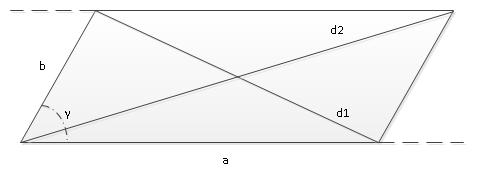homechevron_rightStudychevron_rightMathchevron_rightGeometry

# The diagonals of a parallelogram

Calculator computes the diagonals of a parallelogram and adjancent angles from side lengths and angle.This content is licensed under Creative Commons Attribution/Share-Alike License 3.0 (Unported). That means you may freely redistribute or modify this content under the same license conditions and must attribute the original author by placing a hyperlink from your site to this work https://planetcalc.com/1149/. Also, please do not modify any references to the original work (if any) contained in this content.This calculator computes the diagonals of a parallelogram and adjancent angles from side lengths and angle between sides.

It is done with the help of law of cosines

Check the picture. First, we use law of cosines to find out d1, then we find second angle of parallelogram, which is , then we again use law of cosines to find out d2. Remaining angles are found from the same law of cosines. After that we find diagonal intersection angles using the fact that sum of triangle angles is 180.#### The polygon diagonals of a parallelogram

°
Digits after the decimal point: 2
Diagonal d1

Diagonal d2

Second parallelogram angle

Angle between d1 and d2

Angle between a and d1

Angle between b and d1

Angle between a and d2

Angle between b and d2PLANETCALC, The diagonals of a parallelogram